# Texas Go Math Grade 3 Lesson 13.1 Answer Key Divide by 6

Refer to our Texas Go Math Grade 3 Answer Key Pdf to score good marks in the exams. Test yourself by practicing the problems from Texas Go Math Grade 3 Lesson 13.1 Answer Key Divide by 6.

## Texas Go Math Grade 3 Lesson 13.1 Answer Key Divide by 6

Essential Question
What strategies can you use to divide by 6?

Unlock the problem
Ms. Sing needs to buy 24 juice boxes for the class picnic. Juice boxes come in packs of 6. How many packs does Ms. Sing need to buy?

• Circle the number that tells you how many juice boxes come in a pack.
• How can you use the information to solve the problem?

One Way
Make equal groups.

• Draw 24 counters.
• Circle as many groups of 6 as you can.
• Count the number of groups.

There are ___ groups of 6.
So, Ms. Sing needs to buy ___ packs of juice boxes.
You can write ___ ÷ ___ or.

Math Talk
Mathematical Processes
If you divided the 24 counters into groups of 4, how many groups would there be? Explain how you know.

Other Ways
A. Write a related multiplication fact.B. Use factors to find 24 ÷ 6.
The factors of 6 are 3 and 2.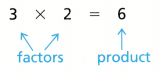To divide by 6, use the factors.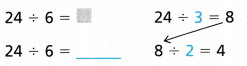Share and Show

Question 1.
Continue making equal groups to find 18 ÷ 6.Math Talk
Mathematical Processes
Explain how you could use factors to find 18 ÷ 6.

Find the unknown factor and quotient.

Question 2.
6 × __ = 12 12 ÷ 6 = ___

Find the quotient.

Question 3.
42 ÷ 6 = ___

Problem Solving

Question 4.
k = 54 ÷ 6
k = __

Question 5.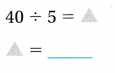Question 6.
0 ÷ 6 = s
s= ___

Question 7.
60 ÷ 10 = m
m = ___

Algebra Find the unknown number.

Question 8.
42 ÷ ____ = 7

Question 9.
24 ÷ ____ = 8

Question 10.
16 ÷ ___ = 4

Question 11.
32 ÷ __ = 4

Problem Solving

Question 12.
What’s the Error? Mary has 36 stickers to give to 6 friends. She says she can give each friend only 5 stickers. Use a division equation to describe Mary’s error.

Question 13.
Analyze Cody baked 12 muffins. He keeps 6 muffins. How many muffins can he give to each of his 6 friends if each friend gets the same number of muffins?

Question 14.
H.O.T. Multi-Step Derek bought 2 boxes of fruit snacks. There were 12 in each box. If he eats 6 fruit snacks each day how many days will the fruit snacks last? Explain.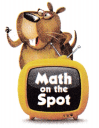Question 15.
H.O.T. Pose a Problem Write and solve a word problem for the strip diagram.Fill in the bubble for the correct answer choice. You may use a division strategy to help you solve.

Question 16.
One day, 6 friends explore 18 blocks of a town. The friends divide the blocks equally. How many blocks does each friend explore?
(A) 4
(B) 2
(C) 1
(D) 3

Question 17.
Reasoning Mr. Brooks has 36 students in his gym class. He makes 6 teams. If each team has the same number of students, how many students are on each team?
(A) 6
(B) 8
(C) 4
(D) 5

Question 18.
Multi-Step Sandy bakes 18 pies. She keeps 2 of the pies. She sells the rest of the pies to 4 people at a bake sale.
If each person buys the same number of pies, how many pies does Sandy sell to each person?
(A) 16
(B) 4
(C) 9
(D) 8

Texas Test Prep

Question 19.
Each picnic table at a park can seat up to 6 people. How many tables will 48 people fill?
(A) 6
(B) 8
(C) 7
(D) 9

### Texas Go Math Grade 3 Lesson 13.1 Homework and Practice Answer Key

Find the unknown number.

Question 1.
z = 48 ÷ 6
z = ___

Question 2.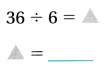Question 3.
60 ÷ 6 = p
p = ___

Question 4.
18 ÷ ____ = 3

Question 5.
35 ÷ __ = 7

Question 6.
28 ÷ ___ = 7

Question 7.
12 ÷ __ = 2

Question 8.
6 ÷ __ = 1

Question 9.
15 ÷ __ = 5

Problem Solving

Question 10.
Chantell buys 36 apples. She keeps 6 for herself. How many apples can she give to each of 6 neighbors if each person gets the same number of apples?

Question 11.
Andre has 21 tomato plants. He gives 3 to a friend, then plants the rest in 6 equal rows. How many tomato plants are in each row?

Question 12.
Jake has 42 baseball cards that he wants to share equally with himself and 5 friends. How many cards will each person get? Write a division sentence to solve the problem.

Question 13.
Write and solve a word problem for the strip diagram.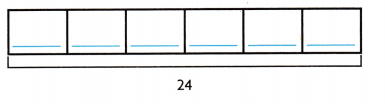Lesson Check

Question 14.
There are 54 people going on arafting trip. Each raft holds 6 people. How many rafts are needed for the trip?
(A) 8
(B) 6
(C) 9
(D) 7

Question 15.
There are 24 puppies at the dog show. They compete in equal groups of 4. How many puppies are needed for the trip? each group?
(A) 20
(B) 28
(C) 6
(D) 8

Question 16.
There are 30 scouts marching in a parade. The scout troop marches in equal rows of 5. How many rows of scouts are in the parade?
(A) 6
(B) 7
(C) 5
(D) 8

Question 17.
A vegetable stand has 42 baskets of different vegetables. The baskets are set up in 6 equal rows. How many baskets are in each row?
(A) 5
(B) 7
(C) 6
(D) 8

Question 18.
Multi-Step Tanya bakes 10 trays of 6 muffins. She keeps 1 tray for herself and gives an equal number away to 6 friends. How many muffins does Tanya share with each friend?
(A) 9
(B) 20
(C) 6
(D) 16